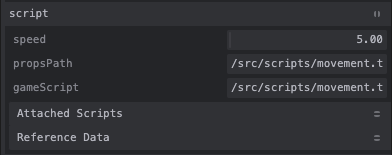# User ​

In this part of the tutorial we will make the user paddle.

## Setup ​

1. Copy the cube gameObject and rename it to userPaddle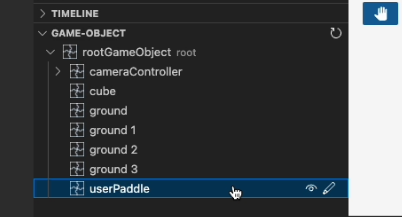2. Move the userPaddle to [0,8,15.5]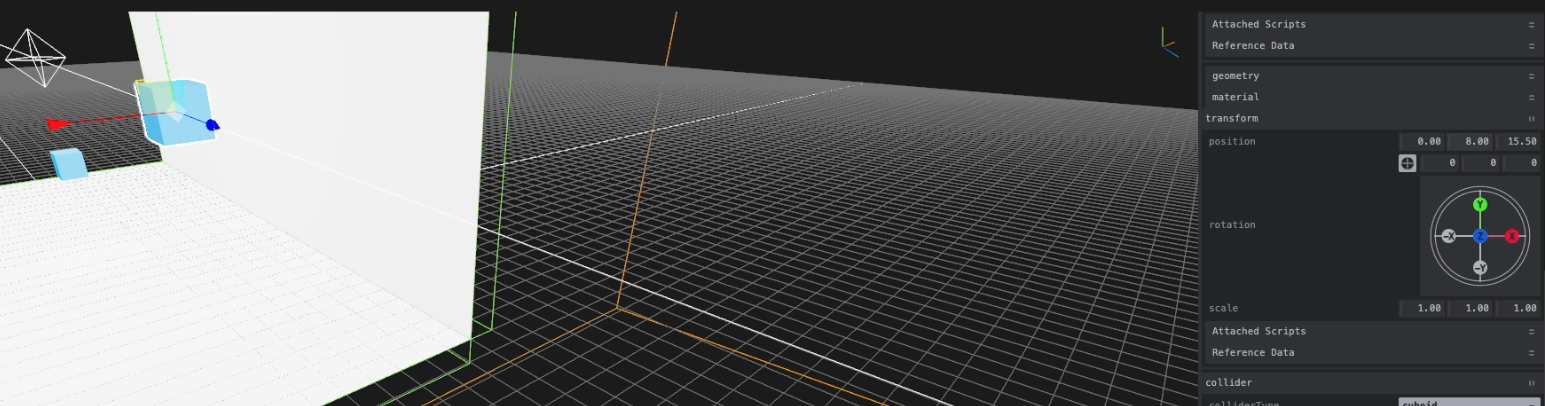3. Scale the userPaddle to [5,5,1]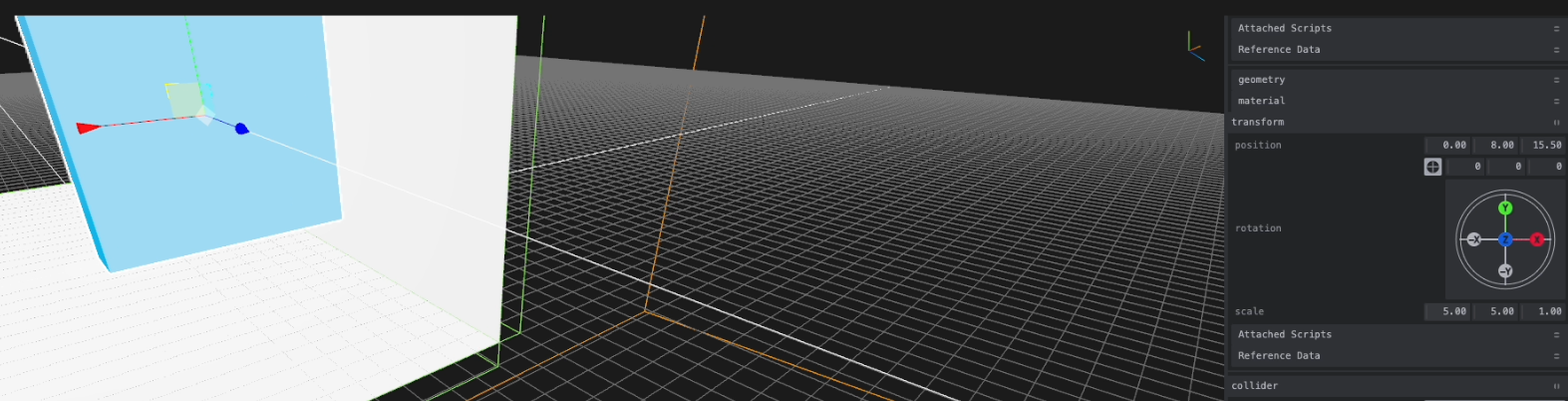4. Change the color of the userPaddle to green by updating the material inside the mesh component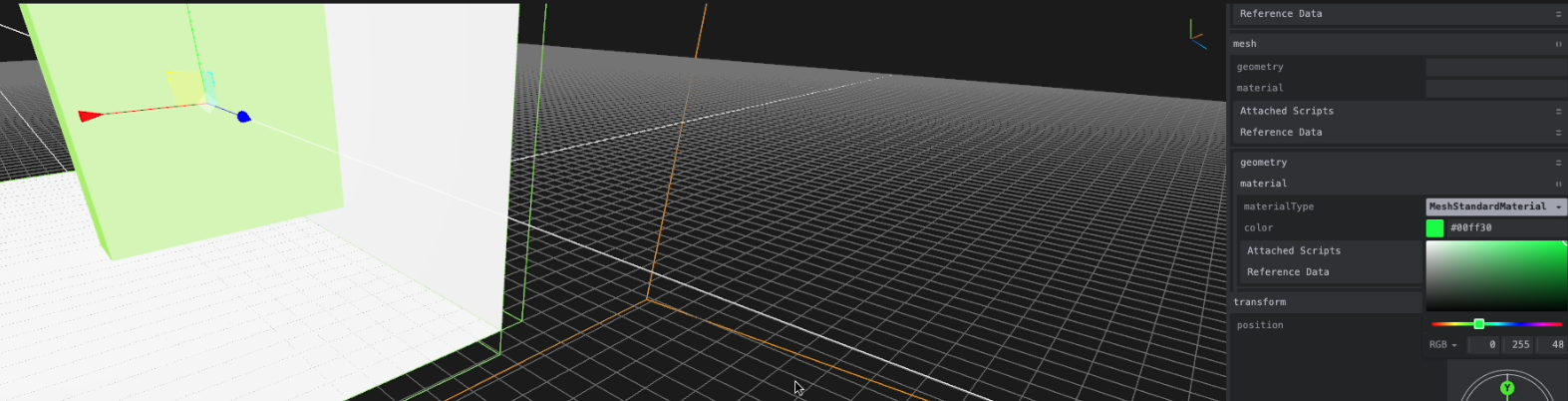5. Change the rigidBodyType in the rigidBody component to KinematicVelocityBased. This is done because we want the paddle to not be affected by collisions (hence kinematic) and we will control it by changing its velocity(hence velocity based)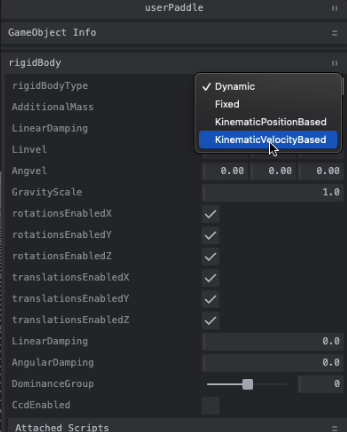## Logic ​

1. We add the logic in `<projectRoot>/src/scripts/movement.ts` file.
TypeScript
``````export const props = { // exposed in the component editor
speed: 5,//speed of movement
};

export const init = (game, gameObject, {props}) => {
let horizontal = 0;
let vertical = 0;

if(!props.speed)props.speed = 5;//use default speed if speed was not changed

const updateLinVel = () => {// function to update linear velocity
const rb = gameObject.components.rigidBody;
if(!rb)return;
rb.setLinvel({ x: horizontal* props.speed, y: vertical*props.speed, z: 0 }, true);
};

if (e.repeat) { return } // return if this is not the first time this event is fired for this keypress
switch(e.code){// update the direction of motion
case 'KeyA':
horizontal -= 1;
break;
case 'KeyD':
horizontal += 1;
break;
case 'KeyS':
vertical -= 1;
break;
case 'KeyW':
vertical += 1;
break;
};
updateLinVel();// update linear velocity
});

switch(e.code){// update the direction of motion
case 'KeyA':
horizontal += 1;
break;
case 'KeyD':
horizontal -= 1;
break;
case 'KeyS':
vertical += 1;
break;
case 'KeyW':
vertical -= 1;
break;
};
updateLinVel();// update linear velocity
});
}``````

the exported `props` are visible in the component editor.

1. Add this script to the userPaddle